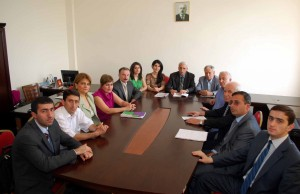﻿ BAKU STATE UNIVERSITY

The chair of Theory of functions and functional analysisThe chair of theory of functions and functional analysis was founded in 1939 by Academician A.I.Huseynov, who had directed it till 1965.

In 1965-1992 prof. A.Sh. Habibzade and prof. O.A.Valiyev were the  heads of the chair 1992-1996. From 1996 up to now the chair is headed by d.p.-m.s., prof. A.M.Akhmedov.

In the chair the bachelors study next branches of sciences: theory of functions of real variables; functional analysis and theory of functions of complex variables and related special courses; the masters study the basic and special courses: spectral theory of operators, complex analysis and applications, operator-differential equations, linear equation in Banach space, generalized functions and  functional spaces.

More than one hundred articles, tens of monographs, text-books, training appliances and methodical instructions of teaching of branches were published in the chair.

Collagorators:

Akhmedov A.M. - Head of the department, professor, doctor of physical and mathematical sciences

Mamedkhanov C.I.- professor, doctor of physical and mathematical sciences

Tagiyeva M.A. - associate professor, candidate of physical and mathematical sciences

Babayev R.M. - associate professor, candidate of physical and mathematical sciences

Rzayev E.A. - associate professor, candidate of physical and mathematical sciences

Gasimov T.B. - associate professor, candidate of physical and mathematical sciences

Ismailov M.I.- associate professor, candidate of physical and mathematical sciences

Babayeva S.F.- associate professor, Ph. D. in mathematical sciences

Asadov T.B.- associate professor, Ph. D. in mathematical sciences

Mansimova H.S.- lecturer, candidate of physical and mathematical sciences

Aslanbeyova G.F.- senior  laboratory assistant

The taught  main disciplines

Bachelors

Main directions of speculations:

Theory  of functions of real variables

Functional  analysis

Theory  of functions of complex variables

The taught  disciplines

Main:

Theory  of functions of real variables

Functional  analysis

Theory  of functions of complex variables

Selected:

Selected chapters of theory of functions

Selected chapters of functional  analysis

The taught  disciplines for masters

Main:

Spectral  theory of operators

Complex  analysis and applications

Operator-differential equations

Selected:

Linear  equation in Banach space

Generalized  functions

Functional  spaces